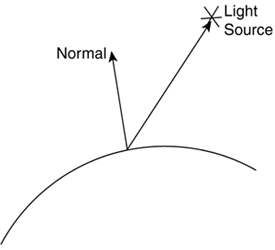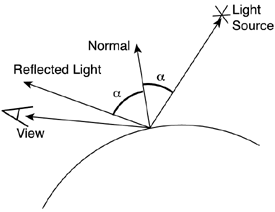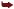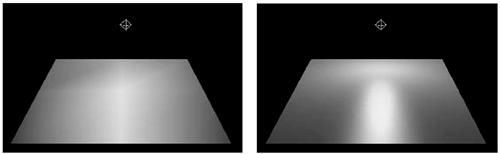# Real-World Illumination

Before talking about illumination algorithms for real-time games, let's stop and do an overview of how real lighting works. This will provide us with a solid foundation from which software algorithms can be derived.

Light is both an electromagnetic wave and a stream of particles (called photons), which travel through space and interact with different surfaces. In a vacuum, light travels at exactly 299,792,458 meters per second, or approximately 300 million meters/second. Speed decreases as the medium becomes more resistive to light. Light travels slower in the air, even slower inside water, and so on. As an example, a team from Harvard and Stanford University was able to slow down a light beam to as little as 17 meters per second (that's around 40 miles per hour) by making it cross an ultracold sodium atom gas.

Light emanates from surfaces when their atoms are energized by heat, electricity, or a host of chemical reactions. As atoms receive energy, their electrons use that incoming energy to move from their natural orbit to higher orbits, much like traffic on the fast lane of a highway. Sooner or later, these atoms will fall back to the normal orbit, releasing a packet of energy in the process. This packet is what we commonly call one photon. A photon has a fixed wavelength and frequency depending on the type of orbital change. The frequency determines the light color and also the amount of energy the light beam will transport. On the lower energy side, we have red light with a frequency of 430GHz. On the opposite end is violet light, which has a frequency of 730GHz. Some colors, such as white, are not related to a fixed frequency, but are achieved by the sum of different frequencies in a light beam in an interference pattern. But remember that light occupies a tiny fraction of the wave frequency spectrum. Radio waves have lower energy than any visible light, whereas x-rays and gamma rays transport more energy.

As light travels through space it might hit an object, and part of it might bounce back. When a hit occurs, photons hit the electrons of the object, energize them, and eventually they fall back again, emitting new photons. So really what you see is a secondary light ray that can in fact be of a different frequency (and thus color) than the first one because of the orbital change in the object. The way objects absorb some frequencies and thus generate light in a specific color pattern makes us see the color in objects. Thus, we can see light from a light source or from an object absorbing light selectively. But notice how both cases are internally similar: An object is just a secondary light source.

When light hits an object, the energy beam is divided into three main components. One part is absorbed by the object, usually increasing its energy levels in the form of heat. You feel that component whenever you lie on a sunny beach. A second component bounces off the surface and generates a reflected ray of light. That's what we see in a mirror or any reflective material. A third light beam enters the object (usually changing its speed due to the variation of density between both mediums) and travels through it. This phenomenon is called refraction or transmission, and the best example is light entering the sea. The change of speed makes the light rays bend, sometimes making us think objects immersed in the medium are broken (like a straw in a glass viewed sideways).

By understanding the preceding explanation you can model most light phenomena in the real world. Shadows, for example, are nothing but the occlusion of light rays by an opaque object, which in turn makes a region (the shadow volume) appear darker. The glitter on the crest of waves is just the reflection of the sunlight and only happens when the wave's orientation allows for a perfect reflection. Even the light concentration phenomena such as a hotspot caused by a lens or the patterns in a swimming pool can be explained. As the rays refract entering the water, they bend. Because many bent rays converge on a small area, it ends up receiving lots of energy, and appears burnt. Unfortunately, the nature of light cannot be directly transported to a computer. As with most atom-level phenomena, the amount of data required for a real-world simulation is prohibitive by today's standards. Computing any scene would require shooting billions of photons and tracing them around the scene to model their behavior accurately. That's what some offline rendering algorithms, such as ray tracing or radiosity, do. They are used in commercial renderers, but take anything from minutes to days to render a single frame.

#### A Simple Rendering Equation

For the sake of game programming, we will now explore some computational methods that simulate light interaction. We will start with a relatively straightforward algorithm used in many graphics applications and games. Later in this chapter, we will explore more involved solutions like the Bidirectional Reflectance Distribution Function (BRDF). But to begin with, we will use a model that computes lighting in a point as the result of three components:

• Ambient. Light that scatters in all directions and provides a base lighting to the scene.

• Diffuse. Light reflected from surfaces in all directions. The amount of reflected light is proportional to the angle of incidence of the light striking the surface. This component is viewpoint independent.

• Specular. Light reflected on a surface along the mirror direction, which accounts for pure reflection between the object and the light source. Like all mirrors, the intensity is view dependent.

Here is the global lighting equation for such a model:

` Color = Ka*ambientColor + Kd*diffuseColor*(N dot L) + Ks*specularColor*(R dot V)shininess `

The equation has three major components, one for ambient, one for diffuse, and one for specular. Let's review each.

Ka, Kd, and Ks perform a breakdown of the lighting components. Different materials have different proportions of each one, but when added, these components should be 1. Typical values are Ka=0.2, Kd=0.5, and Ks=0.3, for example.

The three colors (ambientColor, diffuseColor, and specularColor) are RGB triplets specifying the colors of the three components. They can be computed using different criteria. The ambientColor, for example, is usually white or some subtle color that has to do with daylight color: pinkish in the evening, and so on. The reason for using white light as ambient is that, generally speaking, we can assume that the scene has many light waves of different wavelengths, so they result in white light when combined. Diffuse and specular color are dependent on the object's color and light source color. The specular component, for example, is usually initialized with the light source color, whereas the diffuse color must take both the surface and light colors into consideration. Intuitively, a white ball illuminated with blue light does not look pure white, nor does it look pure blue. Thus, using the following technique is common:

` ambientColor = white diffuseColor = surfaceColor * lightColor specularColor = lightColor `

Notice that this is an approximation. Nature does not have an ambient component per se, and multiplying surface and light source colors for diffuse color is somewhat wrong. But results are very good and can be computed in real time.

Now, let's take a look at the rest of the equation. The diffuse component is scaled by (N dot L), where N is the object's normal, and L is the vector from the point being shaded to the light source. Assuming both vectors are normalized, this means diffuse contribution is total whenever the light falls on the object parallel to its normal thus, in a perfectly vertical way. This configuration is showcased in Figure 17.1.

##### Figure 17.1. Normal and light vector explained.All light is then bounced back, and the diffuse contribution is maximal. Then, the specular contribution is scaled by (R dot V), where R is the light's reflected vector, and V is the viewing vector. This configuration is showcased in Figure 17.2

##### Figure 17.2. Normal, light, reflected light, and view vector illustrated.Intuitively, light is reflected off the surface as in a mirror, and if we are angularly close to that mirror (that's what R dot V means), we see a hotspot. The only problem is computing R and V. Here are the equations:

` V = vector from the point being shaded to our position R = 2*N*(N dot L) - L `

The formulation for R can take advantage of N dot L, which was already computed for the diffuse component.

Also, note how we add an exponent to the equation. Intuitively, polished objects show smaller, more focused highlights, so the shininess parameter helps us model that.

Here is a generalized equation that adds support for multiple sources and takes attenuation into consideration:

` Color = Ka*ambientColor + S(1/(kC+kL*di+kQdi2))*(Kd*diffuseColori* (N dot Li) + Ks*specularColori*(Ridot V)shininess) `

Notice that a global, light-source independent ambient contribution is added to the sum of the individual contribution of each lamp in the scene. Then, each lamp has a diffuse and specular component. I have added the i subindex to represent those values that must be computed per light, such as colors and reflection vectors.

Now, a note on attenuation factors: In the real world, light is absorbed by the distance squared. This is very well suited for the large distances present in the real world, but gives strange results in computer graphics. So, both OpenGL and DirectX use a slightly different model, where attenuation is a general quadratic equation in the form:

` attenuation=1/(kC+kL*di+kQdi2) `

In the equation, di is the distance between the point being shaded and the light source. Now all we have to do is tune the kC, kL, and kQ parameters to reach the results we want. A constant attenuation, for example, would be expressed as (kC!=0, kL=kQ=0). On the opposite end, a quadratic equation that mimics the real world would be achieved by (kC=0, kL=0, kQ!=0). And the very popular linear attenuation used by many games is achieved by kC=0, kL!=0, kQ=0.

A word of warning on these kinds of equations: These are ideal models that do not take many factors into consideration. Shadows, for example, need to incorporate the scene geometry into the equation. If a point is in shadow from a light source, its diffuse and specular components would either be eliminated completely (for opaque occluders) or scaled by the opacity value (if the object causing the shadow is semitransparent). We will talk about shadows later in the chapter in their own section.

#### Per-Vertex and Per-Pixel Lighting

The first way to generate convincing illumination effects on a computer game is to use per-vertex lighting. This type of lighting is computed at the vertices of the geometry only and interpolated in between. Thus, to illuminate a triangle we would compute a lighting equation at each one of the three vertices. Then, the hardware would use these three values to interpolate the illumination of the entire triangle.

The rendering equation from the previous section is a popular choice because it can be implemented easily. In fact, variants of that equation are used internally by both OpenGL and DirectX renderers.

But per-vertex lighting is only computed as vertices. So what will happen to a large wall represented by only two triangles? Imagine that we place a light source right in the middle of the quad, far away from the four vertices. In the real world, the light source would create a very bright hotspot in the center of the wall, but because we do not have vertices there, per-vertex illumination will look completely wrong (see Figure 17.3). We need to either refine the mesh (which will definitely impact the bus and GPU) or find a better way to shade.

##### Figure 17.3. Per-vertex (left) versus per-pixel (right) lighting.This is where per-pixel shading kicks in. This lighting mode does not compute lighting at the vertices only, but in any pixel in between, so illumination has higher resolution and quality. Techniques such as light mapping (explained in the next section) or fragment shaders (explained in Chapter 21, "Procedural Techniques") are used to compute per-pixel lighting.

But if per-vertex lighting is what you are looking for, there's two options. You can either precompute lighting colors and store them as per-vertex colors, or you can leave this task to OpenGL or DirectX lighting engines. The first approach has the advantage of being faster because no computations are done in the real-time loop (lighting is a costly equation). On the other hand, the specular contribution cannot be precomputed because it is view dependent. The second option takes advantage of current-generation GPUs, which all support hardware lighting and transforms.

You can find complete examples of how lighting works in Appendix B, "OpenGL," and Appendix C, "Direct3D."Core Techniques and Algorithms in Game Programming2003
ISBN: N/A
EAN: N/A
Year: 2004
Pages: 261